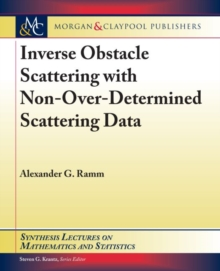Supporting your high street Find out how »
• My AccountInverse Obstacle Scattering with Non-Over-Determined Scattering Data Hardback

Part of the Synthesis Lectures on Mathematics and Statistics series

Description

The inverse obstacle scattering problem consists of finding the unknown surface of a body (obstacle) from the scattering A(B;a;k), where A(B;a;k) is the scattering amplitude, B;a E S2 is the direction of the scattered, incident wave, respectively, S2 is the unit sphere in the R3 and k > 0 is the modulus of the wave vector.

The scattering data is called non-over-determined if its dimensionality is the same as the one of the unknown object.

By the dimensionality one understands the minimal number of variables of a function describing the data or an object.

In an inverse obstacle scattering problem this number is 2, and an example of non-over-determined data is A(B) := A(B;a0;k0).

By sub-index 0 a fixed value of a variable is denoted. It is proved in this book that the data A(B), known for all B in an open subset of S2, determines uniquely the surface S and the boundary condition on S.

This condition can be the Dirichlet, or the Neumann, or the impedance type. The above uniqueness theorem is of principal importance because the non-over-determined data are the minimal data determining uniquely the unknown S.

There were no such results in the literature, therefore the need for this book arose.

This book contains a self-contained proof of the existence and uniqueness of the scattering solution for rough surfaces.

Information

• Format: Hardback
• Pages: 69 pages
• Publisher: Morgan & Claypool Publishers
• Publication Date:
• Category: Numerical analysis
• ISBN: 9781681735900

Other Formats

£54.50

on all orders

Pick up orders

from local bookshops

£34.50

£38.95

£58.50

£138.00

£117.30

£69.50

£69.25

£88.95

£87.95

£34.50

£59.95